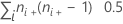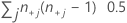# Methods and formulas for measures of concordance for Cross Tabulation and Chi-Square

Select the method or formula of your choice.

## Number of concordant and discordant pairs

A pair is concordant if the observation ranking higher on variable X also ranks higher on variable Y. The pair is discordant if the observation ranking higher on X ranks lower on Y. The pair is tied if the subjects have the same classification on X and/or Y.

### Notation

TermDescription
nij observations in the cell corresponding to the ith row and jth column

## Gamma

Goodman and Kruskal's gamma is a measure of association between the ordinal variables. Perfect association exists when |γ| = 1. If X and Y are independent, γ = 0.

### Notation

TermDescription
C number of concordant pairs = Σi<kΣj<l nij nkl
D number of discordant pairs = Σi<kΣj>l nij nkl
nij observations in the cell corresponding to ith row and jth column

## Somers' D

Somers' D measures the strength and direction of the relationship between two ordinal variables.

### Formula

With Y as the response variable:

With X as the response variable:

### Notation

TermDescription
TX number of pairs tied on X =TY number of pairs tied on Y =C number of concordant pairs
D number of discordant pairs
ni+ number of observations in the ith row
n+j number of observations in the jth column
nij observations in the cell corresponding to the ith row and jth column
n++ total number of observations

## Kendall's Tau-b

Kendall's tau-b, like gamma, measures the association between ordinal variables. One strength of Kendall's tau-b as a measure of association is that it accounts for tied pairs in its calculation. Gamma has a problem with tied pairs, so gamma almost always shows a higher association than tau-b. The tau-b values range from -1.0 to 1.0.

### Notation

TermDescription
TX number of pairs tied on X = Σi ni+ (ni+- 1) 0.5
TY number of pairs tied on Y = Σj n+j (n+j- 1) 0.5
C number of concordant pairs = Σi<kΣj<l nij nkl
D number of discordant pairs = Σi<kΣj>l nij nkl
ni+ number of observations in the ith row
n+j number of observations in the jth column
nij observations in the cell corresponding to ith row and jth column
n++ total number of observations
By using this site you agree to the use of cookies for analytics and personalized content.  Read our policy